Project tutorial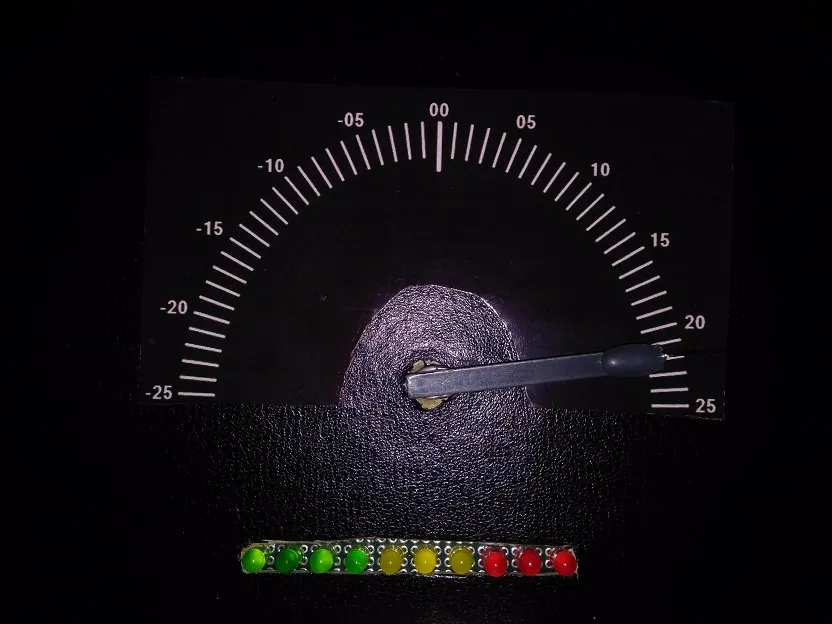# Vintage Bench-Top DC Hybrid Voltmeter © GPL3+

With a DC range of -25V to +25V, a voltmeter combining analog needle & digital LEDs to represent integer & fractional voltages respectively.

• 1,635 views
• 10 respects

## Components and supplies

×1
 Servo Motor
×1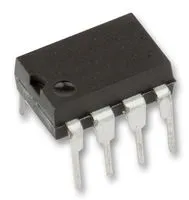Texas Instruments General Purpose Dual Op-Amp
×1
 SparkFun .1 uF Capacitor
×1
 Resistor 10 k
×1
 Resistor 1M
×2
 10 K Variable Multi Turn Pot
×1
 16 Mhz Xtal
×1×1
 Old Desktop Calendar
×1
 4AA Power Pack
×1
 SparkFun Jumper Wire M/F
×1
×1
 SparkFun 100uF Capacitor Electrolyte
×1
 10 uF Capacitor Electrolyte
×1
 SparkFun RGBY Mix LEDs
×1

## Necessary tools and machines

 Hot glue gun

## Apps and online services

 Arduino IDE

### Intro

Once upon a time before the digital era, voltmeters were made with springs, needle pointer, EM coils and burden loads. Nowadays, many kids don't know what those looked like!

This is the story of an analog-looking hybrid voltmeter using digital stuff!

Don't wanna read ? Watch then ......

### Features

• Capable of measuring voltage drop across any resistor in live circuit
• Measure negative voltage
• Active range -25 volts DC to +25 volts DC
• Accuracy of +/- 0.1 volts for voltage source
• Accuracy of 90% for voltage drop measured across resistor
• Battery powered
• Artwork for desktop
• High input resistance voltage divider
• Floating ground is buffered to source/sink current

### Build

The enclosure is made from old desktop calendar. The needle movement is done by servo motor and decimal is presented by 10 LEDs. An appropriate sized slit and a hole is made on the inner walls of the calendar and the servo motor and LEDs are hot glue mounted.

It can measure from negative 25 to positive 25 volts with a resolution of 0.1 volts. The dial is made in MSWord using wordArt, the screenshot us transferred to Paint, inverted to get the dial. Next printed on photo paper with Inkjet printer.

Next, the control circuit is built on a breadboard, the breadboard is placed inside the calender's cleavage.

Finally, the dial is cut from the photo paper and using gum, it is placed on the front side.

### Program

Programming can be done in two ways. The program is written in Arduino IDE.

Method 1

Removing the actual Atmega328P from Arduino UNO board and then placing the new Atmega328P with Arduino bootloader in it. Then uploading the sketch provided, then removing the chip back to breadboard.

Method 2

Using a USB to FTDI (Serial) Module. In this method the module upload the code over serial to the bootloaded UNO chip.

Tutorial here.

### Application

This is not a replacement of regular voltmeter. It is mainly useful when multiple voltages are required to be monitored at once.

It can measure voltage difference/ drop across a resistor but there is a problem known as loading effect. An 500k by 10k resistor voltage divider network is used to attenuate larger voltages to fit ADC range (0 to 1.1 volts). This resistor divider is in parallel to the external circuit and can change the actual voltage by loading it.

Basically it's best for measuring power supply voltages, battery voltages and voltage drop across smaller (few K) resistors.

### Negative Voltage Measurement Explained!

The negative voltage measurement is done with the help of a generic Opamp and some passives using a trick.

There is a function in Arduino called analogReference(INTERNAL); which will put a constant 1.1 V ( may vary from between 1.06 to 1.13 from chip to chip) on AREF pin of Uno/ATmega328P . Once this function is called all ADC conversion will happen between Gnd and AREF Volt rail.

Gnd to AREF or Gnd to Vcc range ADC conversion is known as Single ended Conversion, which requires that there the ADC measurement is done with respect to common ground ( Arduino ground ). This is not suitable for Negative Voltage Measurement.

The trick is to use one of the two points between which the voltage is measured of any external circuit ( could be one terminal of a battery or one point of a resistor) connected to 1/2 of AREF voltage. This way the other point's potential could be either greater ( in case of + ) or less ( in case of -- ) than this 1/2 AREF point, so a difference between two points can be measured.

The AREF pin can't drive load ( i.e. a voltage divider resistor ). So, this voltage is buffered with an Opamp. Buffering means, forwarding the same signal/voltage with more current driving capability and protecting the incoming signal from getting overloaded/reduced. Then the buffered output is voltage divided using variable resistor to get 1/2 AREF voltage ( in this case 0.544 V ). This half voltage is buffered again with another Opamp, Then the second buffered voltage is connected to one point of the external circuit. The other point is also connected to the external voltage. Between these two points there is voltage divider to scale down the measurement voltage. The mid point of the divider is sampled by the Arduino ADC.

The circuit looks like this -

'

This method has two disadvantage :

• ADC resolution drops 1 MSB, which means 10 bit ADC will become 9 bit.
• Any voltage measured on the internal circuit is not possible using that ADC pin

## Code

##### SketchArduino
```#include <Servo.h>
# define AREF 1.08

float volt=0.0;
int offset=-3;
Servo needle;

void setup()
{
Servo_init();
LED_init();
needle_calibrate();
LED_test();
}

void loop()
{
for (int i=0;i<512;i++)
{
volt = volt+ 20*(x-512)*4.9*(AREF/2)/1023;

}
volt = volt/512;

if (volt<.09 & volt > 0.0)
{ volt = 0.0; } // rel (zeroing)

volt = volt - 0.03;// offset correction
if ( volt < 0.0 )
volt = volt - 0.19;

int pos;
pos = map(volt,-25,25,0,180);
needle.write(pos+offset);
LED_meter(volt); // glows LEDs according to fraction
delay(500);
volt=0.0;
}

{
analogReference(INTERNAL);  // set 1.1 v on AREF pin

}

void Servo_init(void)
{
needle.attach(2);  // attaches the servo on pin 2

}

void LED_init (void)
{
pinMode(3,1);
pinMode(4,1);
pinMode(5,1);
pinMode(6,1);
pinMode(7,1);
pinMode(8,1);
pinMode(9,1);
pinMode(10,1);
pinMode(11,1);
pinMode(12,1);
}

void needle_calibrate (void)
{

needle.write(0);
delay(1000);
needle.write(90);
delay(1000);
needle.write(180);
delay(1000);

}

void measure_volt (void)
{

}

void correct_volt (float v)
{

}

void volt_meter (void)
{

}

void LED_meter(float v)
{
int z = (v*10);
int y = v;
int x = z%y;
if (x==9)
{
digitalWrite (3,1);
digitalWrite (4,0);
digitalWrite (5,0);
digitalWrite (6,0);
digitalWrite (7,0);
digitalWrite (8,0);
digitalWrite (9,0);
digitalWrite (10,0);
digitalWrite (11,0);
digitalWrite (12,0);
}
if (x==8)
{
digitalWrite (3,1);
digitalWrite (4,1);
digitalWrite (5,0);
digitalWrite (6,0);
digitalWrite (7,0);
digitalWrite (8,0);
digitalWrite (9,0);
digitalWrite (10,0);
digitalWrite (11,0);
digitalWrite (12,0);
}

if (x==7)
{
digitalWrite (3,1);
digitalWrite (4,1);
digitalWrite (5,1);
digitalWrite (6,0);
digitalWrite (7,0);
digitalWrite (8,0);
digitalWrite (9,0);
digitalWrite (10,0);
digitalWrite (11,0);
digitalWrite (12,0);
}

if (x==6)
{
digitalWrite (3,1);
digitalWrite (4,1);
digitalWrite (5,1);
digitalWrite (6,1);
digitalWrite (7,0);
digitalWrite (8,0);
digitalWrite (9,0);
digitalWrite (10,0);
digitalWrite (11,0);
digitalWrite (12,0);
}
if (x==5)
{
digitalWrite (3,1);
digitalWrite (4,1);
digitalWrite (5,1);
digitalWrite (6,1);
digitalWrite (7,1);
digitalWrite (8,0);
digitalWrite (9,0);
digitalWrite (10,0);
digitalWrite (11,0);
digitalWrite (12,0);
}
if (x==4)
{
digitalWrite (3,1);
digitalWrite (4,1);
digitalWrite (5,1);
digitalWrite (6,1);
digitalWrite (7,1);
digitalWrite (8,1);
digitalWrite (9,0);
digitalWrite (10,0);
digitalWrite (11,0);
digitalWrite (12,0);
}

if (x==3)
{
digitalWrite (3,1);
digitalWrite (4,1);
digitalWrite (5,1);
digitalWrite (6,1);
digitalWrite (7,1);
digitalWrite (8,1);
digitalWrite (9,1);
digitalWrite (10,0);
digitalWrite (11,0);
digitalWrite (12,0);
}
if (x==2)
{
digitalWrite (3,1);
digitalWrite (4,1);
digitalWrite (5,1);
digitalWrite (6,1);
digitalWrite (7,1);
digitalWrite (8,1);
digitalWrite (9,1);
digitalWrite (10,1);
digitalWrite (11,0);
digitalWrite (12,0);
}
if (x==1)
{
digitalWrite (3,1);
digitalWrite (4,1);
digitalWrite (5,1);
digitalWrite (6,1);
digitalWrite (7,1);
digitalWrite (8,1);
digitalWrite (9,1);
digitalWrite (10,1);
digitalWrite (11,1);
digitalWrite (12,0);
}

}

void LED_test(void)
{

digitalWrite (3,0);
digitalWrite (4,0);
digitalWrite (5,0);
digitalWrite (6,0);
digitalWrite (7,0);
digitalWrite (8,0);
digitalWrite (9,0);
digitalWrite (10,0);
digitalWrite (11,0);
digitalWrite (12,0);
delay(400);
digitalWrite (3,1);
digitalWrite (4,1);
digitalWrite (5,1);
digitalWrite (6,1);
digitalWrite (7,1);
digitalWrite (8,1);
digitalWrite (9,1);
digitalWrite (10,1);
digitalWrite (11,1);
digitalWrite (12,1);
delay(200);

}
```

## Schematics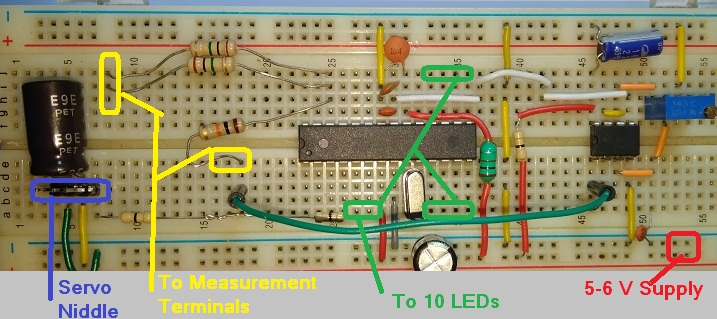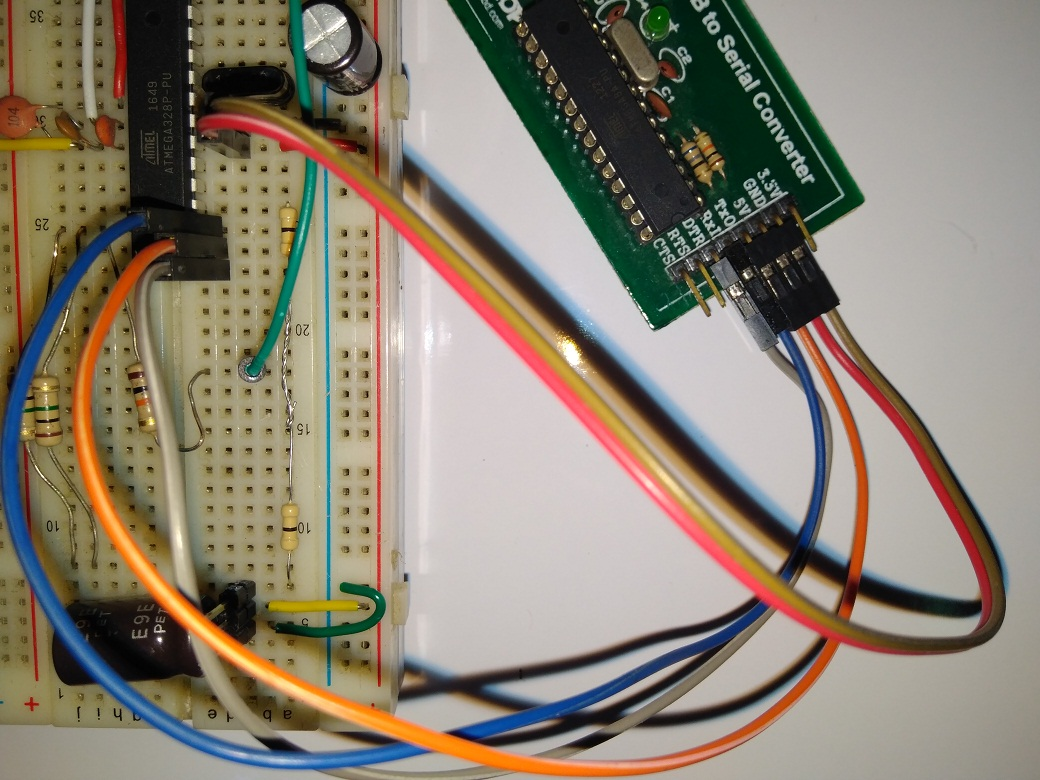vmm_analog_TXfuabjpJe.docx
Black in White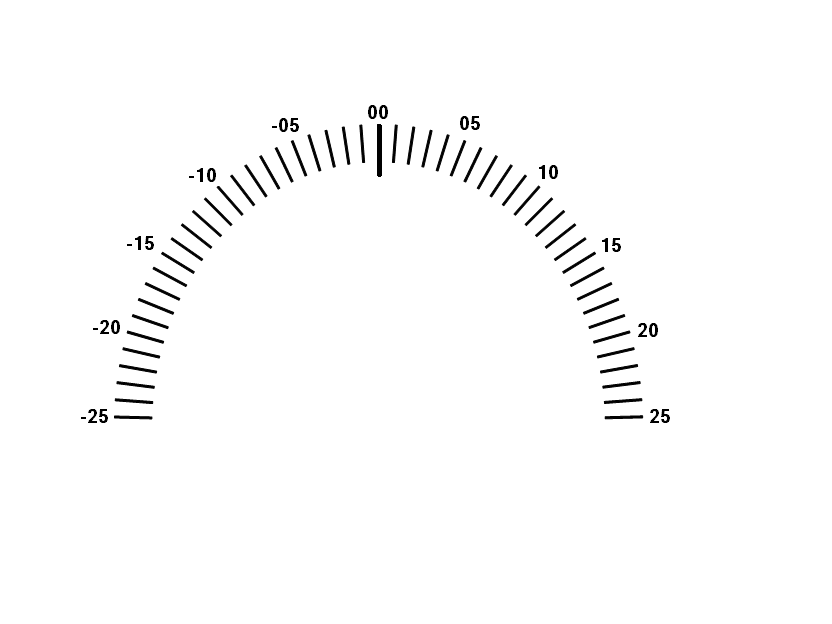White in Black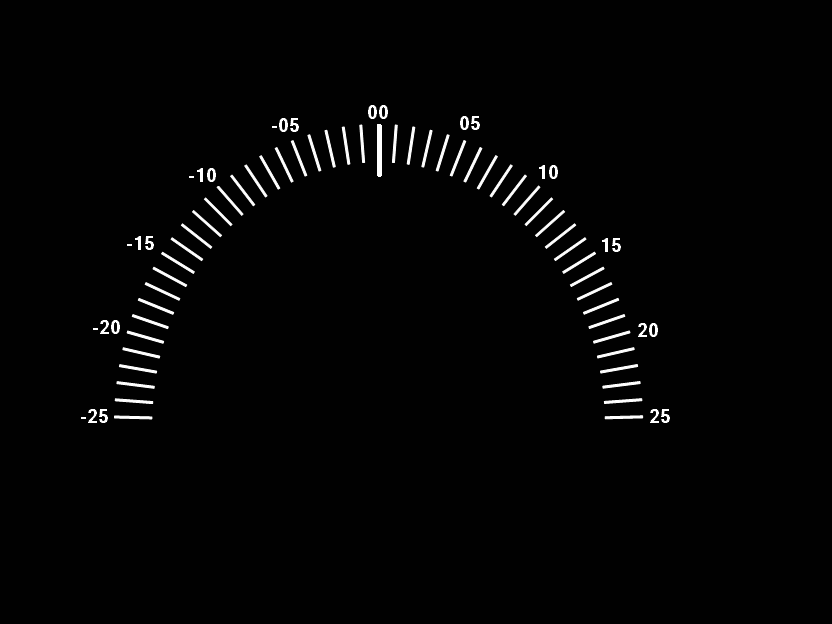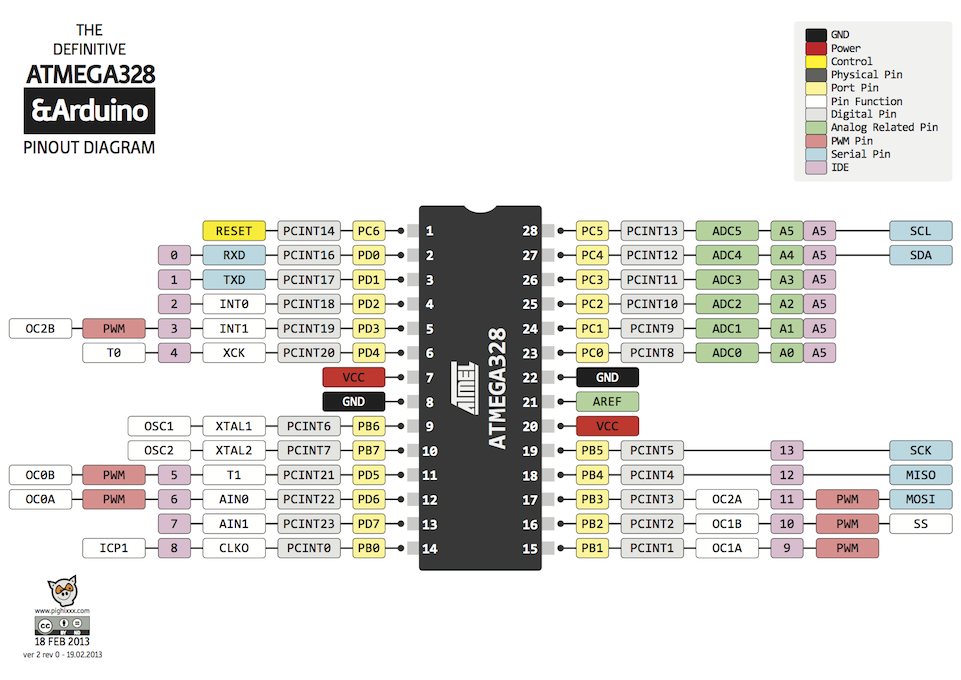#### TFT Graphing: Live History Graphs

Project tutorial by Andrei Florian

• 16,844 views
• 61 respects

#### DIY Voltmeter Using Arduino and Smartphone

Project tutorial by IoT_hobbyist

• 2,816 views
• 30 respects

#### Portable Arduino Temp/Humidity Sensor with LCD

Project tutorial by ThothLoki

• 206,762 views
• 282 respects

#### POV Cylinder with Arduino Due

Project tutorial by Harald Bauer

• 24,424 views
• 78 respects